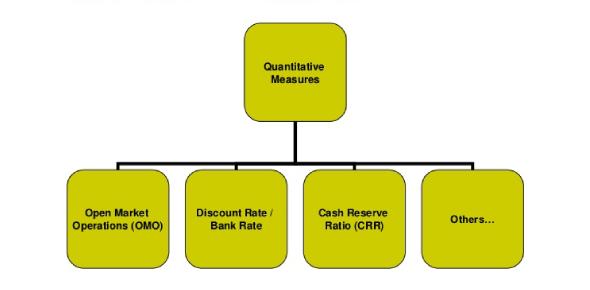# Measures Of Quantitative Assessment (Metrics) Quiz

15 Questions | Attempts: 13160
ShareSettingsMetrics are parameters or measures of quantitative assessment used to measure, compare, or track performance or production. Metrics can help control costs, improve quality, and identify important industry trends. To sign in, type your first name then your last name in the box below. It would help if you viewed the Metrics Tutorial first, then take this Metrics Quiz. All the best!

• 1.
Select the base unit of the metric system for length.
• A.

Liter

• B.

Meter

• C.

Gram

• D.

Kilogram

• E.

Kilometer

• 2.
Select the base unit of the metric system for volume.
• A.

Liter

• B.

Meter

• C.

Gram

• D.

Kilogram

• E.

Kilometer

• 3.
Select the base unit of the metric system for mass.
• A.

Liter

• B.

Meter

• C.

Gram

• D.

Kilogram

• E.

Kilometer

• 4.
Metric units have prefixes that represent multiples of the base. What is the prefix for 1000.0 ?
• A.

Kilo

• B.

Milli

• C.

Centi

• D.

Hecto

• E.

Mega

• 5.
Metric units have prefixes that represent multiples of the base. What is the prefix for 0.000001 ?
• A.

Kilo

• B.

Milli

• C.

Deci

• D.

Mega

• E.

Micro

• 6.
Metric units have prefixes that represent multiples of the base. What is the prefix for 0.01 ?
• A.

Kilo

• B.

Milli

• C.

Centi

• D.

Hecto

• E.

Micro

• 7.
Convert the units 2.7 g into mg.
• A.

0.027

• B.

27

• C.

2700

• D.

0.0027

• E.

27,000,000

• 8.
Convert the units 0.21 km to cm.
• A.

0.21

• B.

21,000

• C.

21

• D.

0.0000021

• E.

21,000,000

• 9.
Convert the units 12.0 ml to l.
• A.

0.12

• B.

12,000

• C.

12,000,000

• D.

0.012

• E.

0.00012

• 10.
Convert 0.00023 to scientific notation. Note: "e" below represents exponent. For example, 2.3 x 10 e5 means 2.3 times 10 to the 5th power.
• A.

0.000023 x 10

• B.

230000 x 10e-1

• C.

2.300 x 10e-6

• D.

2.3 x 10e-4

• E.

2.3 x 10e4

• 11.
Convert 13,796,000 to scientific notation.Note: "e" below represents exponent. For example, 2.3 x 10 e5 means 2.3 times 10 to the 5th power.
• A.

0.000013796 x 10-e1

• B.

13.796 x 10e-2

• C.

1.3796 x 10e-7

• D.

13,796,000 x 10

• E.

1.3796 x 10e7

• 12.
What is the relationship between volume (ml) and mass (g) in the metric system?
• A.

1 ml = 1 g

• B.

1 ml = 1000 g

• C.

1000 ml = 1 g

• D.

100 ml = 1 g

• E.

1 ml = 100 g

• 13.
Convert 23.2 to scientific notation.Note: "e" below represents exponent. For example, 2.3 x 10 e5 means 2.3 times 10 to the 5th power.
• A.

23.2 x 10

• B.

2.32 x 10

• C.

23.2 x 10e-1

• D.

2.32 x 10e-1

• E.

0.0232 x 10e-1

• 14.
The density of water is 1.00. What is the relationship between ml, g and cc?
• A.

1 ml = 10 g = 10 cc

• B.

1 ml = 1 g = 1 cc

• C.

10 ml = 1 g = 10 cc

• D.

100 ml = 1 g = 10 cc

• E.

1 ml = 10 g = 100 cc

• 15.
The density of water is 1.00. Convert 10 ml to g and cc.
• A.

1 g, 10 cc

• B.

0.1 g, 1 cc

• C.

10 g, 10 cc

• D.

100 g, 1000 cc

• E.

1 g, 0.1 cc

## Related TopicsBack to top
×

Wait!
Here's an interesting quiz for you.